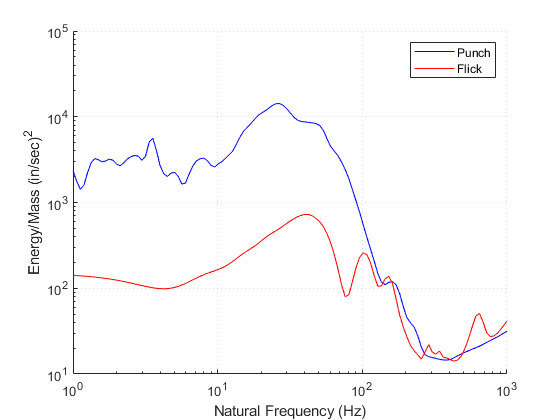# Analyze Data in the VibrationData Toolbox

The VibrationData Toolbox is the MATLAB-compiled Signal Analysis & Structural Dynamics Package by Tom Irvine. This article will go through a few analysis examples. To install the toolbox, download the toolbox from the main site and then read this article.

# Full List of Analysis Functions

The VibrationData Toolbox comes complete with hundreds of different analysis functions, the main GUI screen is shown below that goes through some of the examples.Here is a list of webinars Tom has given to explain the various analysis functions of his VibrationData toolbox. As a reminder, here is the source code if you have a MATLAB license: Vibrationdata Signal Analysis Package.

# PSD

The first example will be to generate a PSD, under Time History.

We'll use the vibration data from the truck bed that was explained and loaded in the help article on loading.  We'll only process the final 20,000 data points.

[time(end-20000:end),Var4(end-20000:end)]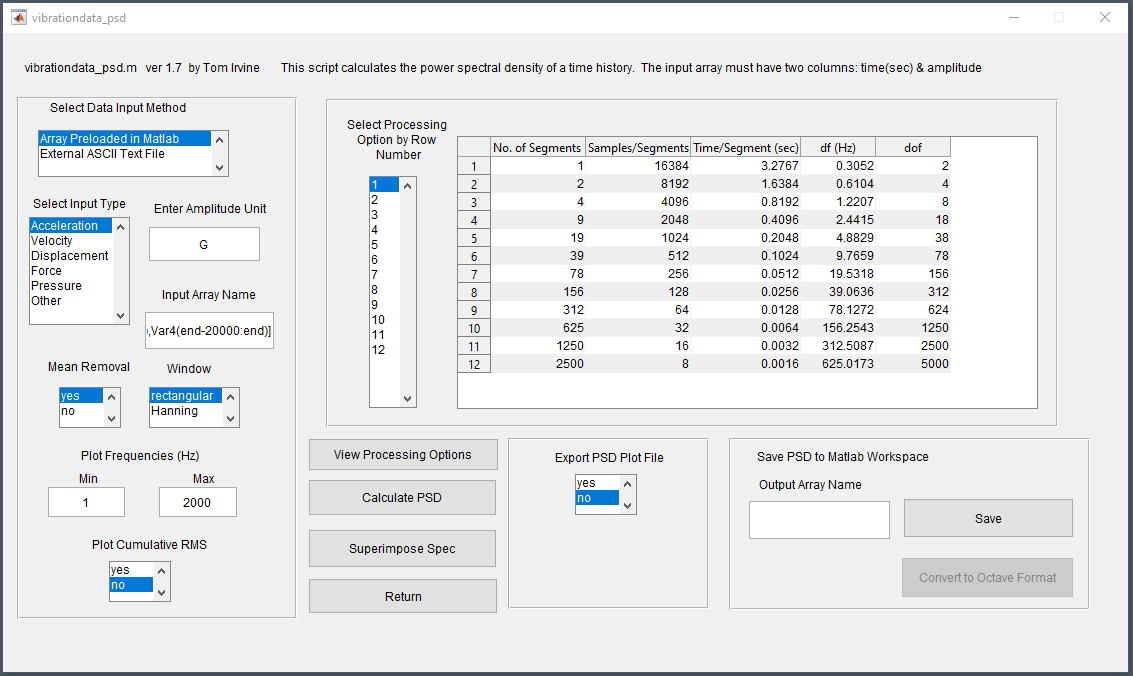The first PSD shown below is for using the standard/first option that has a "bin width" of 0.3 Hz.  You can see this results in a very choppy PSD.  If we go with option 4 that has a bin width of 2.4 Hz, shown in the following image, we get a much cleaner looking PSD.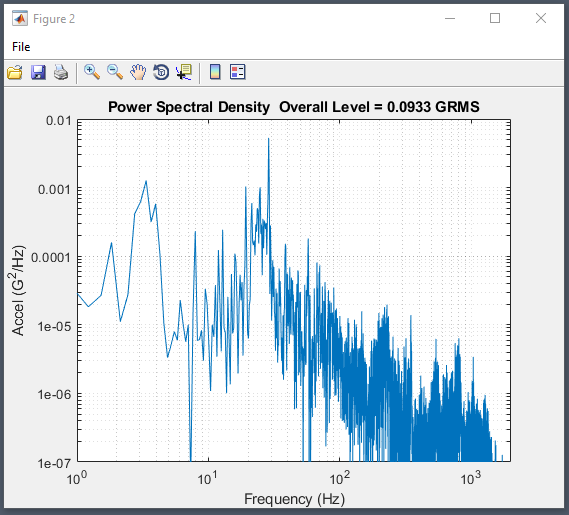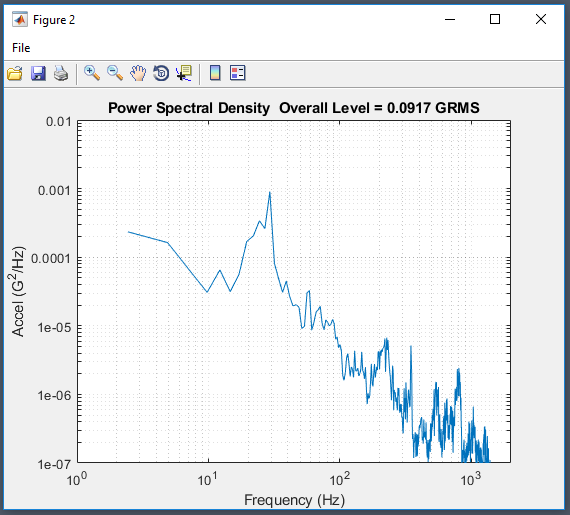Save this PSD to the workspace shown below.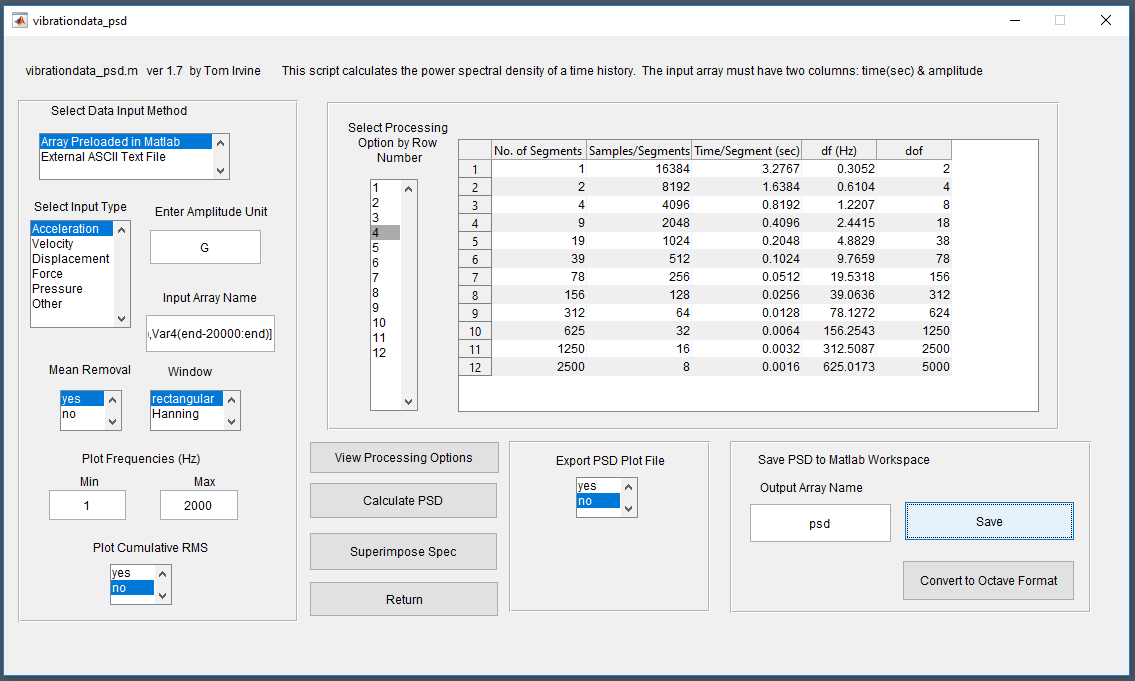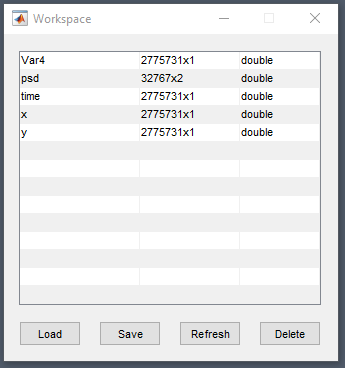# Simplified PSD for Vibration Shaker and/or Test Standard

Often you may need to generate a simplified PSD from experimental data to then use to drive your vibration shaker.  The best way to generate this is to do a PSD Envelope via ERS or FDS. Which uses the energy response or fatigue damage spectrum to calculate that simplified PSD.  To start, select this option under Time History and then the energy response spectrum for this example.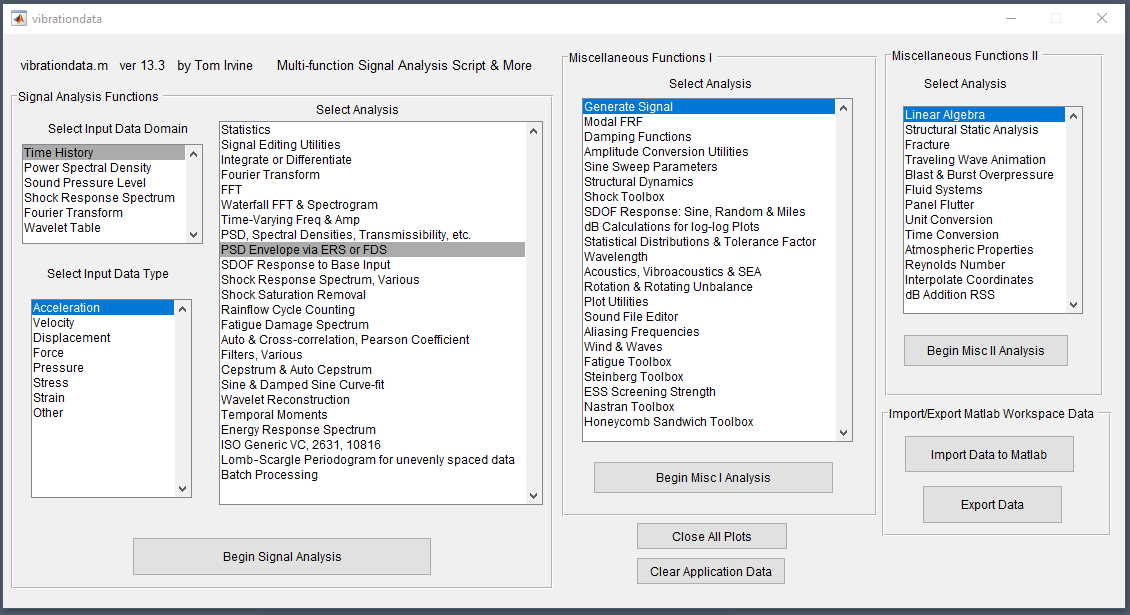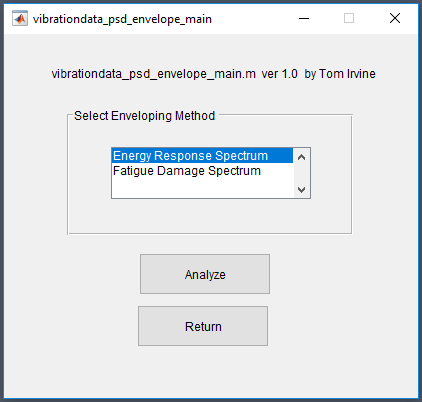Now we define the frequency range (be careful to not set it to be too low which can cause errors, 10 Hz is generally a good rule of thumb unless you have a relatively low sample rate and frequency area of interest).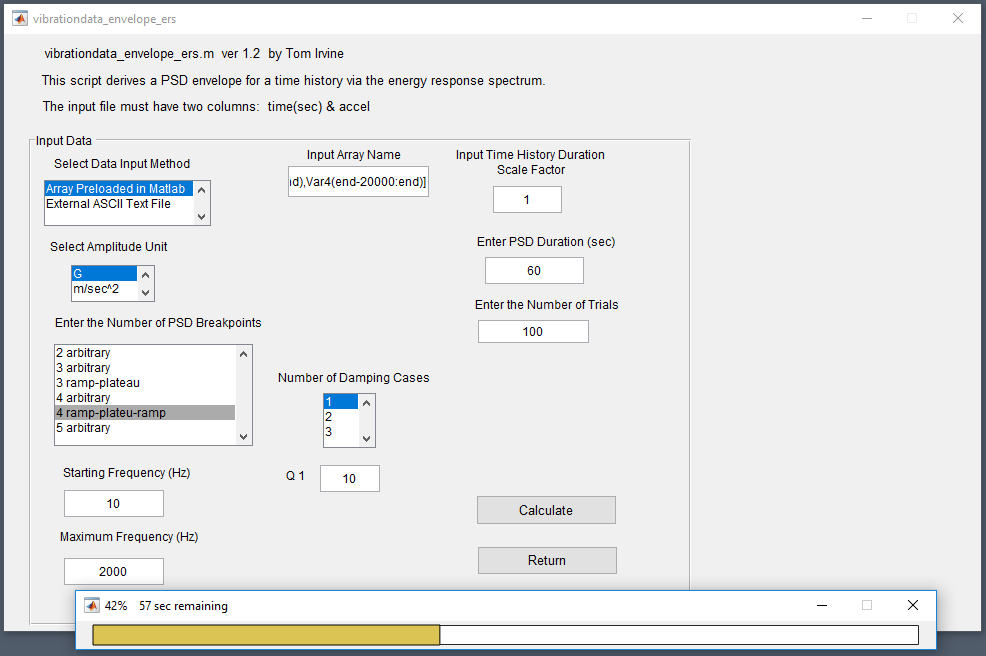You will get a status bar that shows its progress as it simulates 100 different scenarios.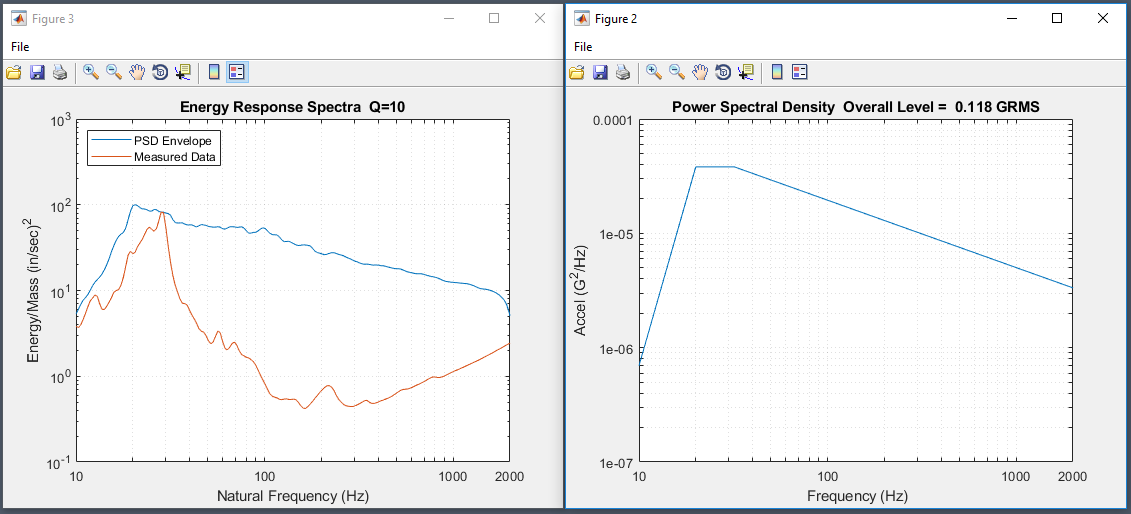You can then save this optimal PSD to the workspace, the coordinates are also provided in the output terminal.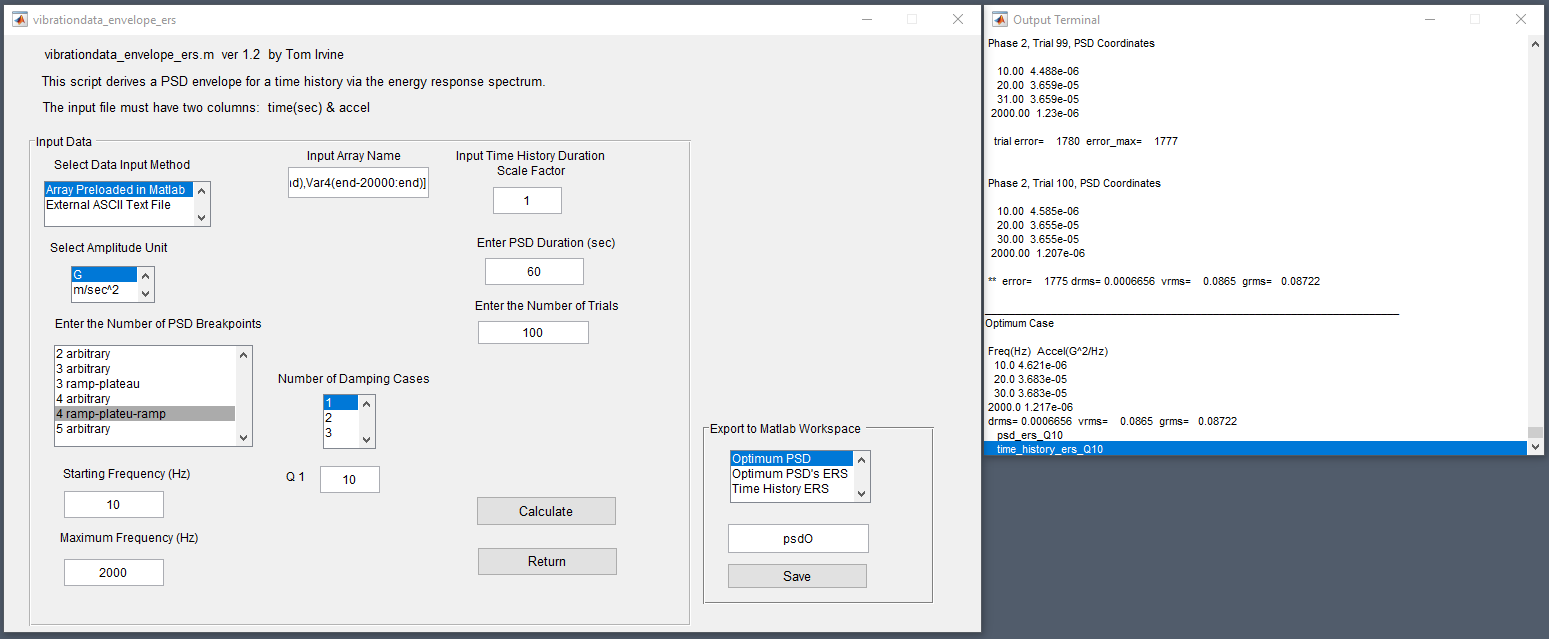Now let's compare to the originally calculated PSD.  This can be done by using the Plot Utilities function and then Two Curves Miscellaneous.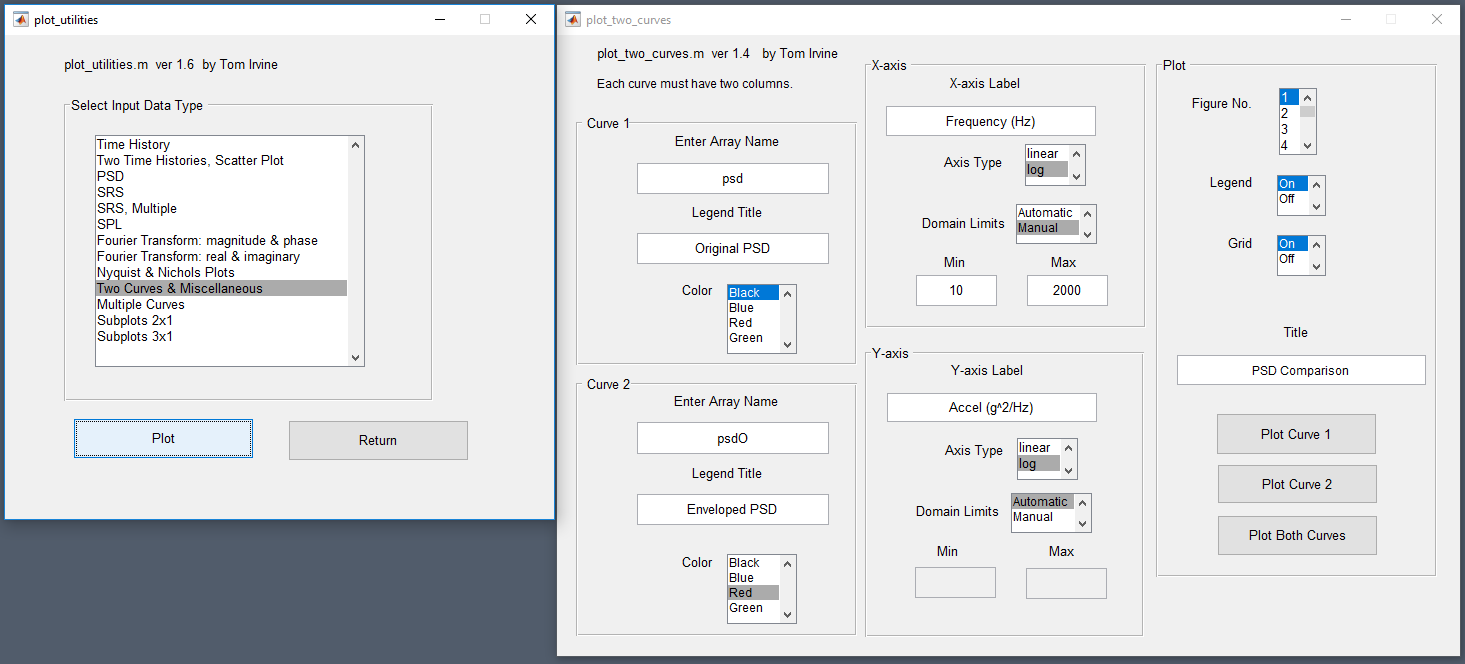The generated plot shows the two spectrograms are close but not as identical as one may imagine.  In certain frequency domains, the enveloped PSD doesn't fully trace the original, but in other frequency domains, it exceeds the original dramatically.  Overall this results in the appropriate amount of energy that you would be enduced into your test article.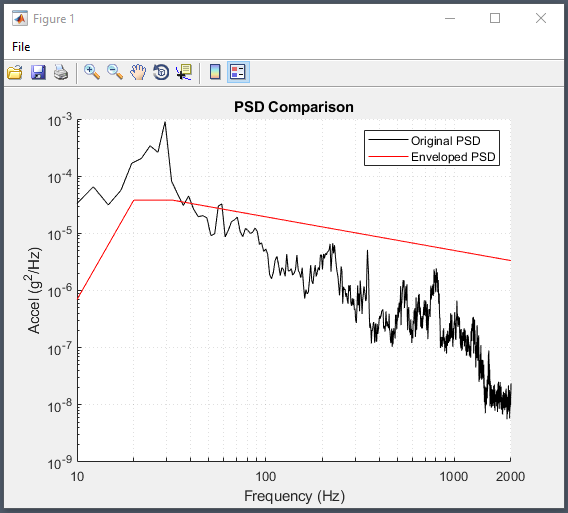# Shock Energy

Acceleration peak and energy are not the same things.  Energy is directly proportional to velocity, not acceleration, meaning that we need take the integral of acceleration.  The VibrationData Toolbox has a great function to calculate the energy response spectrum which will show the energy levels per mass input (simply multiply by the mass of your system) as a function of frequency.  To illustrate this comparison we'll first look at the acceleration levels of a flick and a punch.  This data is shared in our community forum.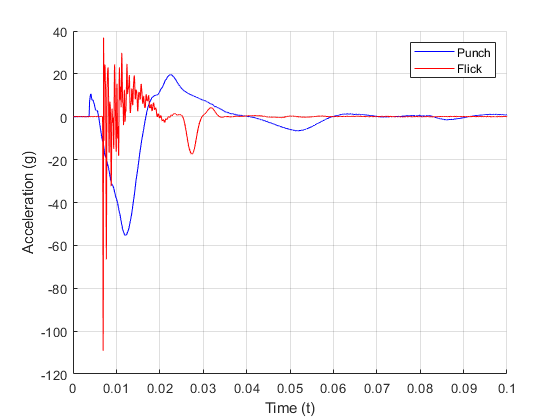The acceleration levels from the flick are much higher, but you can see how quickly they occur and dissipate.  Now let's calculate and compare the energy levels.  To calculate, select the Energy Response Spectrum.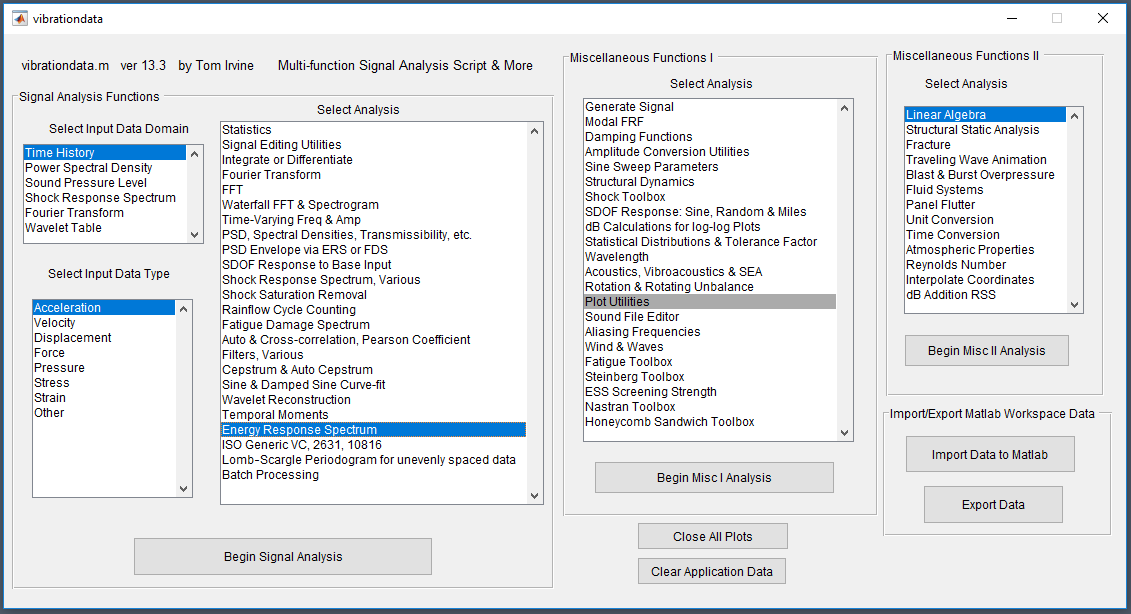The next dialog will ask for the input array and the frequency range.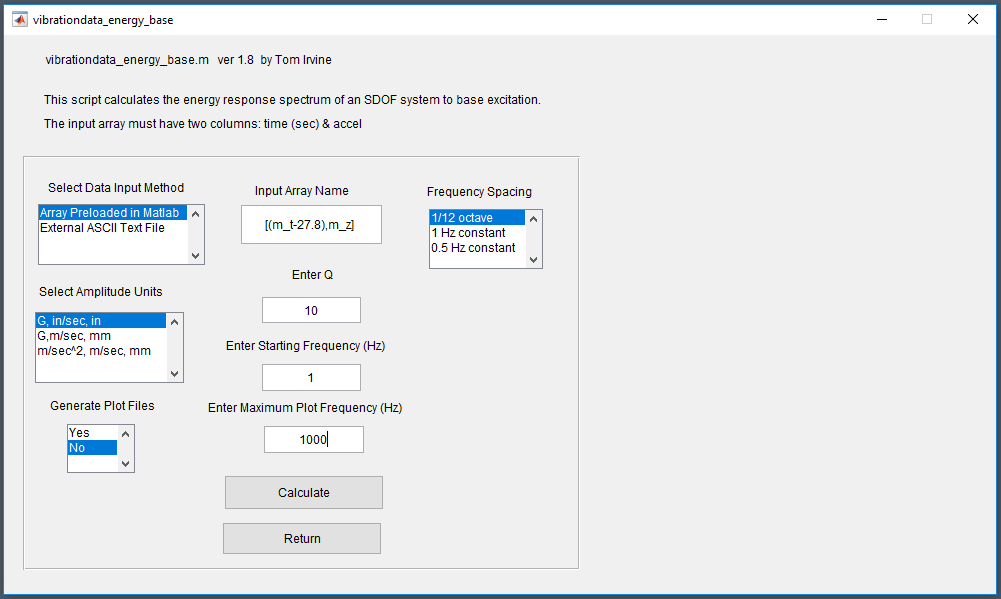This is the output for the punch shock data.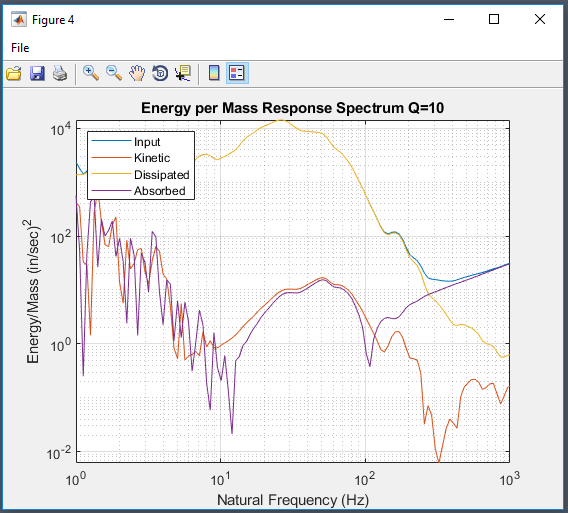And here is the flick energy data.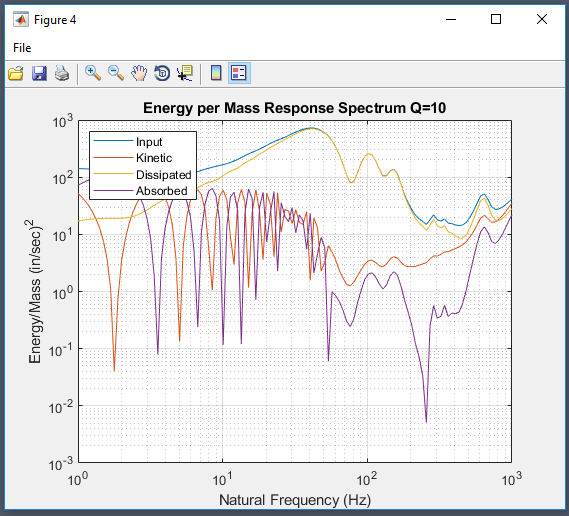You can see the order of magnitude difference in energy levels from the two shock events.  The two energy response spectrums are plotted on one another here.